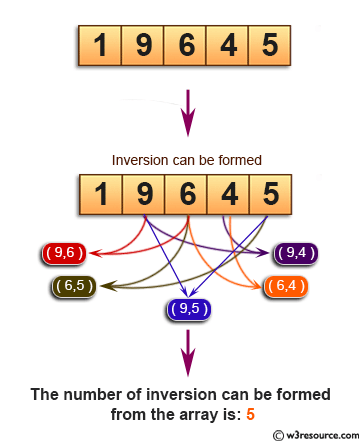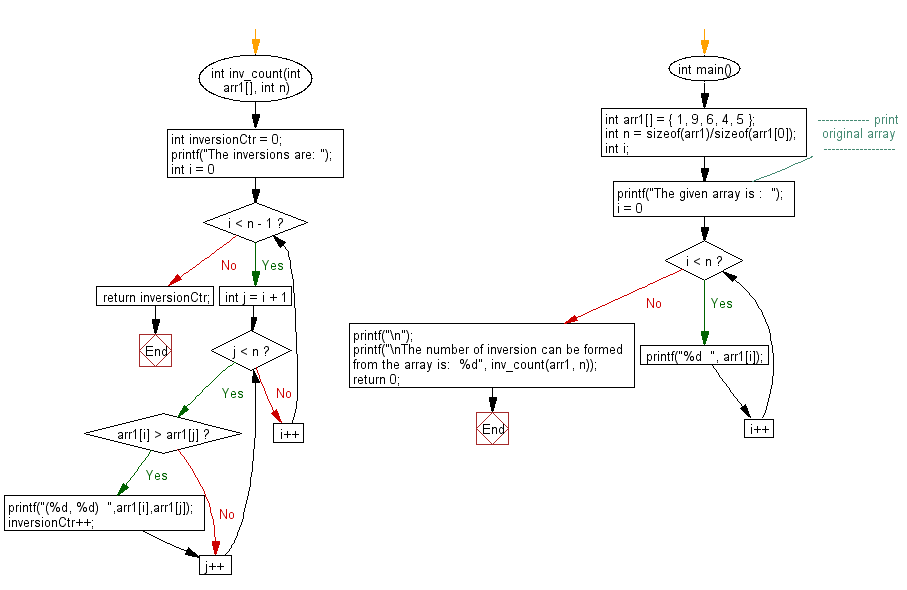﻿ C exercises: Count the number of inversion in a given array - w3resource# C Exercises: Count the number of inversion in a given array

## C Array: Exercise-66 with Solution

Write a program in C to count the number of inversion in a given array.

Pictorial Presentation:Sample Solution:

C Code:

``````#include <stdio.h>
int inv_count(int arr1[], int n)
{
int inversionCtr = 0;
printf("The inversions are: ");
for (int i = 0; i < n - 1; i++)
{
for (int j = i + 1; j < n; j++)
if (arr1[i] > arr1[j])
{
printf("(%d, %d)  ",arr1[i],arr1[j]);
inversionCtr++;
}
}
return inversionCtr;
}

int main()
{
int arr1[] = { 1, 9, 6, 4, 5 };
int n = sizeof(arr1)/sizeof(arr1);
int i;
//------------- print original array ------------------
printf("The given array is :  ");
for(i = 0; i < n; i++)
{
printf("%d  ", arr1[i]);
}
printf("\n");
//------------------------------------------------------
printf("\nThe number of inversion can be formed from the array is:  %d", inv_count(arr1, n));
return 0;
}
```
```

Sample Output:

```The given array is :  1  9  6  4  5
The inversions are: (9, 6)  (9, 4)  (9, 5)  (6, 4)  (6, 5)
The number of inversion can be formed from the array is:  5
```

Flowchart:C Programming Code Editor:

Improve this sample solution and post your code through Disqus.

What is the difficulty level of this exercise?

﻿